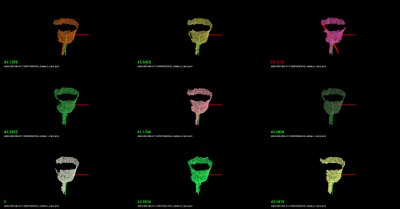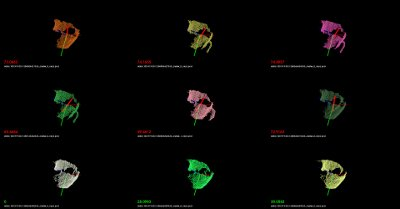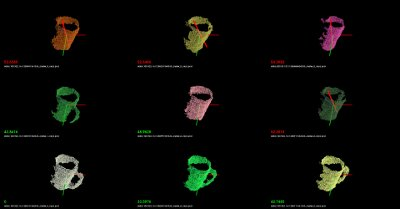# Cluster Recognition and 6DOF Pose Estimation using VFH descriptors

As previously described in Estimating VFH signatures for a set of points, Viewpoint Feature Histograms (VFH) are powerful meta-local descriptors, created for the purpose of recognition and pose estimation for clusters of points. We here refer to a cluster as a collection of 3D points, most of the time representing a particular object or part of a scene, obtained through some segmentation or detection mechanisms (please see Euclidean Cluster Extraction for an example).

Our goal here is not to provide an ultimate recognition tool, but rather a mechanism for obtaining candidates that could potentially be the cluster/object that is searched for, together with its 6DOF pose in space. With this in mind, we will be formulating the recognition problem as a nearest neighbor estimation problem. So given a set of training data, we will use efficient nearest neighbor search structures such as kd-trees and return a set of potential candidates with sorted distances to the query object, rather than an absolute “this is the object that we were searching for” kind of response. The reader can imagine that such a system becomes much more useful as we can explicitly reason about failures (false positives, or true negatives).

For the purpose of this tutorial, the application example could be formulated as follows:

• Training stage:
• given a scene with 1 object that is easily separable as a cluster;
• use a ground-truth system to obtain its pose (see the discussion below);
• rotate around the object or rotate the object with respect to the camera, and compute a VFH descriptor for each view;
• store the views, and build a kd-tree representation.
• Testing stage:
• given a scene with objects that can be separated as individual clusters, first extract the clusters;
• for each cluster, compute a VFH descriptor from the current camera position;
• use the VFH descriptor to search for candidates in the trained kd-tree.

We hope the above makes sense. Basically we’re first going to create the set of objects that we try to later on recognize, and then we will use that to obtain valid candidates for objects in the scene.

A good example of a ground-truth system could be a simple rotating pan-tilt unit such as the one in the figure below. Placing an object on the unit, and moving it with some increments in both horizontal and vertical, can result in a perfect ground-truth system for small objects. A cheaper solution could be to use a marker-based system (e.g., checkerboard) and rotate the camera/table manually.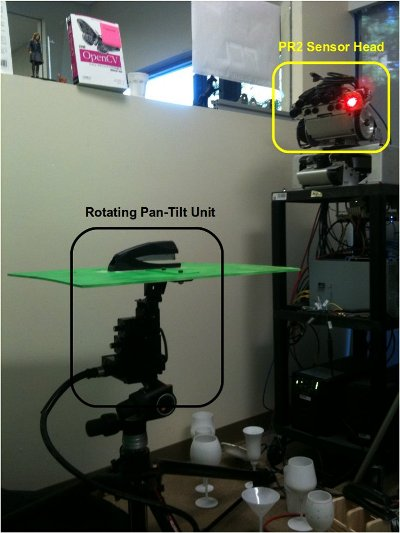Our Kd-Tree implementation of choice for the purpose of this tutorial is of course, FLANN.

# Training

We begin the training by assuming that the objects are already separated as individual clusters (see Euclidean Cluster Extraction), as shown in the figure below: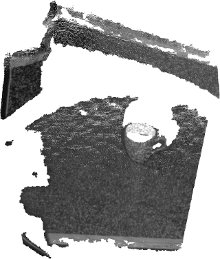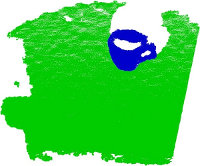Since we’re only trying to cover the explicit training/testing of VFH signatures in this tutorial, we provide a set of datasets already collected at: vfh_recognition_tutorial_data.tbz. The data is a subset of the objects presented in the figure below (left), and look like the point clouds on the right. We used the pan-tilt table shown above to acquire the data.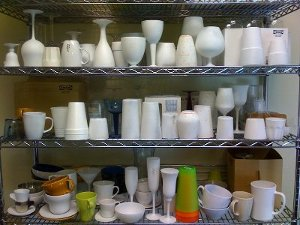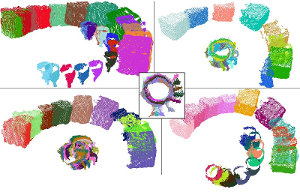Next, copy and paste the following code into your editor and save it as `build_tree.cpp`.

 ``` 1 2 3 4 5 6 7 8 9 10 11 12 13 14 15 16 17 18 19 20 21 22 23 24 25 26 27 28 29 30 31 32 33 34 35 36 37 38 39 40 41 42 43 44 45 46 47 48 49 50 51 52 53 54 55 56 57 58 59 60 61 62 63 64 65 66 67 68 69 70 71 72 73 74 75 76 77 78 79 80 81 82 83 84 85 86 87 88 89 90 91 92 93 94 95 96 97 98 99 100 101 102 103 104 105 106 107 108 109 110 111 112 113 114 115 116 117 118 119 120 121 122 123 124 125 126 127 128 129 130 131 132 133 134 135 136 137``` ```#include #include #include #include #include #include #include #include #include typedef std::pair > vfh_model; /** \brief Loads an n-D histogram file as a VFH signature * \param path the input file name * \param vfh the resultant VFH model */ bool loadHist (const boost::filesystem::path &path, vfh_model &vfh) { int vfh_idx; // Load the file as a PCD try { pcl::PCLPointCloud2 cloud; int version; Eigen::Vector4f origin; Eigen::Quaternionf orientation; pcl::PCDReader r; int type; unsigned int idx; r.readHeader (path.string (), cloud, origin, orientation, version, type, idx); vfh_idx = pcl::getFieldIndex (cloud, "vfh"); if (vfh_idx == -1) return (false); if ((int)cloud.width * cloud.height != 1) return (false); } catch (const pcl::InvalidConversionException&) { return (false); } // Treat the VFH signature as a single Point Cloud pcl::PointCloud point; pcl::io::loadPCDFile (path.string (), point); vfh.second.resize (308); std::vector fields; pcl::getFieldIndex ("vfh", fields); for (std::size_t i = 0; i < fields[vfh_idx].count; ++i) { vfh.second[i] = point.histogram[i]; } vfh.first = path.string (); return (true); } /** \brief Load a set of VFH features that will act as the model (training data) * \param argc the number of arguments (pass from main ()) * \param argv the actual command line arguments (pass from main ()) * \param extension the file extension containing the VFH features * \param models the resultant vector of histogram models */ void loadFeatureModels (const boost::filesystem::path &base_dir, const std::string &extension, std::vector &models) { if (!boost::filesystem::exists (base_dir) && !boost::filesystem::is_directory (base_dir)) return; for (boost::filesystem::directory_iterator it (base_dir); it != boost::filesystem::directory_iterator (); ++it) { if (boost::filesystem::is_directory (it->status ())) { std::stringstream ss; ss << it->path (); pcl::console::print_highlight ("Loading %s (%lu models loaded so far).\n", ss.str ().c_str (), (unsigned long)models.size ()); loadFeatureModels (it->path (), extension, models); } if (boost::filesystem::is_regular_file (it->status ()) && boost::filesystem::extension (it->path ()) == extension) { vfh_model m; if (loadHist (base_dir / it->path ().filename (), m)) models.push_back (m); } } } int main (int argc, char** argv) { if (argc < 2) { PCL_ERROR ("Need at least two parameters! Syntax is: %s [model_directory] [options]\n", argv); return (-1); } std::string extension (".pcd"); transform (extension.begin (), extension.end (), extension.begin (), (int(*)(int))tolower); std::string kdtree_idx_file_name = "kdtree.idx"; std::string training_data_h5_file_name = "training_data.h5"; std::string training_data_list_file_name = "training_data.list"; std::vector models; // Load the model histograms loadFeatureModels (argv, extension, models); pcl::console::print_highlight ("Loaded %d VFH models. Creating training data %s/%s.\n", (int)models.size (), training_data_h5_file_name.c_str (), training_data_list_file_name.c_str ()); // Convert data into FLANN format flann::Matrix data (new float[models.size () * models.second.size ()], models.size (), models.second.size ()); for (std::size_t i = 0; i < data.rows; ++i) for (std::size_t j = 0; j < data.cols; ++j) data[i][j] = models[i].second[j]; // Save data to disk (list of models) flann::save_to_file (data, training_data_h5_file_name, "training_data"); std::ofstream fs; fs.open (training_data_list_file_name.c_str ()); for (std::size_t i = 0; i < models.size (); ++i) fs << models[i].first << "\n"; fs.close (); // Build the tree index and save it to disk pcl::console::print_error ("Building the kdtree index (%s) for %d elements...\n", kdtree_idx_file_name.c_str (), (int)data.rows); flann::Index > index (data, flann::LinearIndexParams ()); //flann::Index > index (data, flann::KDTreeIndexParams (4)); index.buildIndex (); index.save (kdtree_idx_file_name); delete[] data.ptr (); return (0); } ```

In the following paragraphs we will explain what the above code does (or should do). We’ll begin with the `main` function.

We begin by loading a set of feature models from a directory given as the first command line argument (see details for running the example below). The `loadFeatureModels` method does nothing but recursively traverse a set of directories and subdirectories, and loads in all .PCD files it finds. In `loadFeatureModels`, we call `loadHist`, which will attempt to open each PCD file found, read its header, and check whether it contains a VFH signature or not. Together with the VFH signature we also store the PCD file name into a `vfh_model` pair.

Once all VFH features have been loaded, we convert them to FLANN format, using:

```  // Convert data into FLANN format
flann::Matrix<float> data (new float[models.size () * models.second.size ()], models.size (), models.second.size ());

for (std::size_t i = 0; i < data.rows; ++i)
for (std::size_t j = 0; j < data.cols; ++j)
data[i][j] = models[i].second[j];
```

Since we’re lazy, and we want to use this data (and not reload it again by crawling the directory structure in the testing phase), we dump the data to disk:

```  // Save data to disk (list of models)
flann::save_to_file (data, training_data_h5_file_name, "training_data");
std::ofstream fs;
fs.open (training_data_list_file_name.c_str ());
for (std::size_t i = 0; i < models.size (); ++i)
fs << models[i].first << "\n";
fs.close ();
```

Finally, we create the KdTree, and save its structure to disk:

```  pcl::console::print_error ("Building the kdtree index (%s) for %d elements...\n", kdtree_idx_file_name.c_str (), (int)data.rows);
flann::Index<flann::ChiSquareDistance<float> > index (data, flann::LinearIndexParams ());
//flann::Index<flann::ChiSquareDistance<float> > index (data, flann::KDTreeIndexParams (4));
index.buildIndex ();
index.save (kdtree_idx_file_name);
```

Here we will use a `LinearIndex`, which does a brute-force search using a Chi-Square distance metric (see [VFH] for more information). For building a proper kd-tree, comment line 1 and uncomment line 2 in the code snippet above. The most important difference between a LinearIndex and a KDTreeIndex in FLANN is that the KDTree will be much faster, while producing approximate nearest neighbor results, rather than absolute.

So, we’re done with training. To summarize:

1. we crawled a directory structure, looked at all the .PCD files we found, tested them whether they are VFH signatures and loaded them in memory;
2. we converted the data into FLANN format and dumped it to disk;
3. we built a kd-tree structure and dumped it to disk.

# Testing

In the testing phase, we will illustrate how the system works by randomly loading one of the files used in the training phase (feel free to supply your own file here!), and checking the results of the tree.

Begin by copying and pasting the following code into your editor and save it as `nearest_neighbors.cpp`.

 ``` 1 2 3 4 5 6 7 8 9 10 11 12 13 14 15 16 17 18 19 20 21 22 23 24 25 26 27 28 29 30 31 32 33 34 35 36 37 38 39 40 41 42 43 44 45 46 47 48 49 50 51 52 53 54 55 56 57 58 59 60 61 62 63 64 65 66 67 68 69 70 71 72 73 74 75 76 77 78 79 80 81 82 83 84 85 86 87 88 89 90 91 92 93 94 95 96 97 98 99 100 101 102 103 104 105 106 107 108 109 110 111 112 113 114 115 116 117 118 119 120 121 122 123 124 125 126 127 128 129 130 131 132 133 134 135 136 137 138 139 140 141 142 143 144 145 146 147 148 149 150 151 152 153 154 155 156 157 158 159 160 161 162 163 164 165 166 167 168 169 170 171 172 173 174 175 176 177 178 179 180 181 182 183 184 185 186 187 188 189 190 191 192 193 194 195 196 197 198 199 200 201 202 203 204 205 206 207 208 209 210 211 212 213 214 215 216 217 218 219 220 221 222 223 224 225 226 227 228 229 230 231 232 233 234 235 236 237 238 239 240 241 242 243 244 245 246 247 248 249 250 251 252 253 254 255 256 257 258 259 260 261 262 263 264 265 266 267 268 269 270 271 272 273 274``` ```#include #include #include #include #include #include #include #include #include #include #include #include typedef std::pair > vfh_model; /** \brief Loads an n-D histogram file as a VFH signature * \param path the input file name * \param vfh the resultant VFH model */ bool loadHist (const boost::filesystem::path &path, vfh_model &vfh) { int vfh_idx; // Load the file as a PCD try { pcl::PCLPointCloud2 cloud; int version; Eigen::Vector4f origin; Eigen::Quaternionf orientation; pcl::PCDReader r; int type; unsigned int idx; r.readHeader (path.string (), cloud, origin, orientation, version, type, idx); vfh_idx = pcl::getFieldIndex (cloud, "vfh"); if (vfh_idx == -1) return (false); if ((int)cloud.width * cloud.height != 1) return (false); } catch (const pcl::InvalidConversionException&) { return (false); } // Treat the VFH signature as a single Point Cloud pcl::PointCloud point; pcl::io::loadPCDFile (path.string (), point); vfh.second.resize (308); std::vector fields; pcl::getFieldIndex ("vfh", fields); for (std::size_t i = 0; i < fields[vfh_idx].count; ++i) { vfh.second[i] = point.histogram[i]; } vfh.first = path.string (); return (true); } /** \brief Search for the closest k neighbors * \param index the tree * \param model the query model * \param k the number of neighbors to search for * \param indices the resultant neighbor indices * \param distances the resultant neighbor distances */ inline void nearestKSearch (flann::Index > &index, const vfh_model &model, int k, flann::Matrix &indices, flann::Matrix &distances) { // Query point flann::Matrix p = flann::Matrix(new float[model.second.size ()], 1, model.second.size ()); memcpy (&p.ptr (), &model.second, p.cols * p.rows * sizeof (float)); indices = flann::Matrix(new int[k], 1, k); distances = flann::Matrix(new float[k], 1, k); index.knnSearch (p, indices, distances, k, flann::SearchParams (512)); delete[] p.ptr (); } /** \brief Load the list of file model names from an ASCII file * \param models the resultant list of model name * \param filename the input file name */ bool loadFileList (std::vector &models, const std::string &filename) { std::ifstream fs; fs.open (filename.c_str ()); if (!fs.is_open () || fs.fail ()) return (false); std::string line; while (!fs.eof ()) { std::getline (fs, line); if (line.empty ()) continue; vfh_model m; m.first = line; models.push_back (m); } fs.close (); return (true); } int main (int argc, char** argv) { int k = 6; double thresh = DBL_MAX; // No threshold, disabled by default if (argc < 2) { pcl::console::print_error ("Need at least three parameters! Syntax is: %s [options] {kdtree.idx} {training_data.h5} {training_data.list}\n", argv); pcl::console::print_info (" where [options] are: -k = number of nearest neighbors to search for in the tree (default: "); pcl::console::print_value ("%d", k); pcl::console::print_info (")\n"); pcl::console::print_info (" -thresh = maximum distance threshold for a model to be considered VALID (default: "); pcl::console::print_value ("%f", thresh); pcl::console::print_info (")\n\n"); return (-1); } std::string extension (".pcd"); transform (extension.begin (), extension.end (), extension.begin (), (int(*)(int))tolower); // Load the test histogram std::vector pcd_indices = pcl::console::parse_file_extension_argument (argc, argv, ".pcd"); vfh_model histogram; if (!loadHist (argv[pcd_indices.at (0)], histogram)) { pcl::console::print_error ("Cannot load test file %s\n", argv[pcd_indices.at (0)]); return (-1); } pcl::console::parse_argument (argc, argv, "-thresh", thresh); // Search for the k closest matches pcl::console::parse_argument (argc, argv, "-k", k); pcl::console::print_highlight ("Using "); pcl::console::print_value ("%d", k); pcl::console::print_info (" nearest neighbors.\n"); std::string kdtree_idx_file_name = "kdtree.idx"; std::string training_data_h5_file_name = "training_data.h5"; std::string training_data_list_file_name = "training_data.list"; std::vector models; flann::Matrix k_indices; flann::Matrix k_distances; flann::Matrix data; // Check if the data has already been saved to disk if (!boost::filesystem::exists ("training_data.h5") || !boost::filesystem::exists ("training_data.list")) { pcl::console::print_error ("Could not find training data models files %s and %s!\n", training_data_h5_file_name.c_str (), training_data_list_file_name.c_str ()); return (-1); } else { loadFileList (models, training_data_list_file_name); flann::load_from_file (data, training_data_h5_file_name, "training_data"); pcl::console::print_highlight ("Training data found. Loaded %d VFH models from %s/%s.\n", (int)data.rows, training_data_h5_file_name.c_str (), training_data_list_file_name.c_str ()); } // Check if the tree index has already been saved to disk if (!boost::filesystem::exists (kdtree_idx_file_name)) { pcl::console::print_error ("Could not find kd-tree index in file %s!", kdtree_idx_file_name.c_str ()); return (-1); } else { flann::Index > index (data, flann::SavedIndexParams ("kdtree.idx")); index.buildIndex (); nearestKSearch (index, histogram, k, k_indices, k_distances); } // Output the results on screen pcl::console::print_highlight ("The closest %d neighbors for %s are:\n", k, argv[pcd_indices]); for (int i = 0; i < k; ++i) pcl::console::print_info (" %d - %s (%d) with a distance of: %f\n", i, models.at (k_indices[i]).first.c_str (), k_indices[i], k_distances[i]); // Load the results pcl::visualization::PCLVisualizer p (argc, argv, "VFH Cluster Classifier"); int y_s = (int)std::floor (sqrt ((double)k)); int x_s = y_s + (int)std::ceil ((k / (double)y_s) - y_s); double x_step = (double)(1 / (double)x_s); double y_step = (double)(1 / (double)y_s); pcl::console::print_highlight ("Preparing to load "); pcl::console::print_value ("%d", k); pcl::console::print_info (" files ("); pcl::console::print_value ("%d", x_s); pcl::console::print_info ("x"); pcl::console::print_value ("%d", y_s); pcl::console::print_info (" / "); pcl::console::print_value ("%f", x_step); pcl::console::print_info ("x"); pcl::console::print_value ("%f", y_step); pcl::console::print_info (")\n"); int viewport = 0, l = 0, m = 0; for (int i = 0; i < k; ++i) { std::string cloud_name = models.at (k_indices[i]).first; boost::replace_last (cloud_name, "_vfh", ""); p.createViewPort (l * x_step, m * y_step, (l + 1) * x_step, (m + 1) * y_step, viewport); l++; if (l >= x_s) { l = 0; m++; } pcl::PCLPointCloud2 cloud; pcl::console::print_highlight (stderr, "Loading "); pcl::console::print_value (stderr, "%s ", cloud_name.c_str ()); if (pcl::io::loadPCDFile (cloud_name, cloud) == -1) break; // Convert from blob to PointCloud pcl::PointCloud cloud_xyz; pcl::fromPCLPointCloud2 (cloud, cloud_xyz); if (cloud_xyz.size () == 0) break; pcl::console::print_info ("[done, "); pcl::console::print_value ("%zu", static_cast(cloud_xyz.size ())); pcl::console::print_info (" points]\n"); pcl::console::print_info ("Available dimensions: "); pcl::console::print_value ("%s\n", pcl::getFieldsList (cloud).c_str ()); // Demean the cloud Eigen::Vector4f centroid; pcl::compute3DCentroid (cloud_xyz, centroid); pcl::PointCloud::Ptr cloud_xyz_demean (new pcl::PointCloud); pcl::demeanPointCloud (cloud_xyz, centroid, *cloud_xyz_demean); // Add to renderer* p.addPointCloud (cloud_xyz_demean, cloud_name, viewport); // Check if the model found is within our inlier tolerance std::stringstream ss; ss << k_distances[i]; if (k_distances[i] > thresh) { p.addText (ss.str (), 20, 30, 1, 0, 0, ss.str (), viewport); // display the text with red // Create a red line pcl::PointXYZ min_p, max_p; pcl::getMinMax3D (*cloud_xyz_demean, min_p, max_p); std::stringstream line_name; line_name << "line_" << i; p.addLine (min_p, max_p, 1, 0, 0, line_name.str (), viewport); p.setShapeRenderingProperties (pcl::visualization::PCL_VISUALIZER_LINE_WIDTH, 5, line_name.str (), viewport); } else p.addText (ss.str (), 20, 30, 0, 1, 0, ss.str (), viewport); // Increase the font size for the score* p.setShapeRenderingProperties (pcl::visualization::PCL_VISUALIZER_FONT_SIZE, 18, ss.str (), viewport); // Add the cluster name p.addText (cloud_name, 20, 10, cloud_name, viewport); } // Add coordianate systems to all viewports p.addCoordinateSystem (0.1, "global", 0); p.spin (); return (0); } ```

The above code snippet is slightly larger, because we also included some visualization routines and some other “eye candy” stuff.

In lines:

```  std::vector<int> pcd_indices = pcl::console::parse_file_extension_argument (argc, argv, ".pcd");
vfh_model histogram;
{
pcl::console::print_error ("Cannot load test file %s\n", argv[pcd_indices.at (0)]);
return (-1);
}

pcl::console::parse_argument (argc, argv, "-thresh", thresh);
// Search for the k closest matches
pcl::console::parse_argument (argc, argv, "-k", k);
pcl::console::print_highlight ("Using "); pcl::console::print_value ("%d", k); pcl::console::print_info (" nearest neighbors.\n");
```

we load the first given user histogram (and ignore the rest). Then we proceed at checking two command line parameters, namely `-k` which will define how many nearest neighbors to check and display on screen, and `-thresh` which defines a maximum distance metric after which we will start displaying red lines (i.e., crossing) over the k models found on screen (eye candy!).

In lines:

```    loadFileList (models, training_data_list_file_name);
```

we load the training data from disk, together with the list of file names that we previously stored in `build_tree.cpp`. Then, we read the kd-tree and rebuild the index:

```    flann::Index<flann::ChiSquareDistance<float> > index (data, flann::SavedIndexParams ("kdtree.idx"));
index.buildIndex ();
```

Here we need to make sure that we use the exact distance metric (`ChiSquareDistance` in this case), as the one that we used while creating the tree. The most important part of the code comes here:

```    nearestKSearch (index, histogram, k, k_indices, k_distances);
```

Inside `nearestKSearch`, we first convert the query point to FLANN format:

```  flann::Matrix<float> p = flann::Matrix<float>(new float[model.second.size ()], 1, model.second.size ());
memcpy (&p.ptr (), &model.second, p.cols * p.rows * sizeof (float));
```

Followed by obtaining the resultant nearest neighbor indices and distances for the query in:

```  indices = flann::Matrix<int>(new int[k], 1, k);
distances = flann::Matrix<float>(new float[k], 1, k);
index.knnSearch (p, indices, distances, k, flann::SearchParams (512));
```

Lines:

```    index.buildIndex ();
nearestKSearch (index, histogram, k, k_indices, k_distances);
}

// Output the results on screen
pcl::console::print_highlight ("The closest %d neighbors for %s are:\n", k, argv[pcd_indices]);
for (int i = 0; i < k; ++i)
pcl::console::print_info ("    %d - %s (%d) with a distance of: %f\n",
i, models.at (k_indices[i]).first.c_str (), k_indices[i], k_distances[i]);

pcl::visualization::PCLVisualizer p (argc, argv, "VFH Cluster Classifier");
int y_s = (int)std::floor (sqrt ((double)k));
int x_s = y_s + (int)std::ceil ((k / (double)y_s) - y_s);
double x_step = (double)(1 / (double)x_s);
```

create a `PCLVisualizer` object, and sets up a set of different viewports (e.g., splits the screen into different chunks), which will be enabled in:

```    p.createViewPort (l * x_step, m * y_step, (l + 1) * x_step, (m + 1) * y_step, viewport);
```

Using the file names representing the models that we previously obtained in `loadFileList`, we proceed at loading the model file names using:

```    pcl::PCLPointCloud2 cloud;
if (pcl::io::loadPCDFile (cloud_name, cloud) == -1)
break;

// Convert from blob to PointCloud
pcl::PointCloud<pcl::PointXYZ> cloud_xyz;
pcl::fromPCLPointCloud2 (cloud, cloud_xyz);
```

For visualization purposes, we demean the point cloud by computing its centroid and then subtracting it:

```    Eigen::Vector4f centroid;
pcl::compute3DCentroid (cloud_xyz, centroid);
pcl::PointCloud<pcl::PointXYZ>::Ptr cloud_xyz_demean (new pcl::PointCloud<pcl::PointXYZ>);
pcl::demeanPointCloud<pcl::PointXYZ> (cloud_xyz, centroid, *cloud_xyz_demean);
```

Finally we check if the distance obtained by `nearestKSearch` is larger than the user given threshold, and if it is, we display a red line over the cloud that is being rendered in the viewport:

```      // Create a red line
pcl::PointXYZ min_p, max_p;
pcl::getMinMax3D (*cloud_xyz_demean, min_p, max_p);
std::stringstream line_name;
line_name << "line_" << i;
p.addLine (min_p, max_p, 1, 0, 0, line_name.str (), viewport);
p.setShapeRenderingProperties (pcl::visualization::PCL_VISUALIZER_LINE_WIDTH, 5, line_name.str (), viewport);
```

# Compiling and running the code

Create a new `CMakeLists.txt` file, and put the following content into it

 ``` 1 2 3 4 5 6 7 8 9 10 11 12 13 14 15 16 17 18 19 20 21 22``` ```cmake_minimum_required(VERSION 2.8 FATAL_ERROR) project(vfh_cluster_classifier) find_package(PCL 1.2 REQUIRED) include_directories(\${PCL_INCLUDE_DIRS}) link_directories(\${PCL_LIBRARY_DIRS}) add_definitions(\${PCL_DEFINITIONS}) find_package(HDF5 REQUIRED) find_package(FLANN REQUIRED) include_directories(SYSTEM \${HDF5_INCLUDE_DIR} ) add_executable(build_tree build_tree.cpp) target_link_libraries(build_tree \${PCL_LIBRARIES} \${Boost_LIBRARIES} FLANN::FLANN \${HDF5_LIBRARIES}) add_executable(nearest_neighbors nearest_neighbors.cpp) target_link_libraries(nearest_neighbors \${PCL_LIBRARIES} \${Boost_LIBRARIES} FLANN::FLANN \${HDF5_LIBRARIES}) ```

Note

If you are running this tutorial on Windows, you have to install (HDF5 1.8.7 Shared Library). If CMake is not able to find HDF5, you can manually supply the include directory in HDF5_INCLUDE_DIR variable and the full path of hdf5dll.lib in HDF5_hdf5_LIBRARY variable. Make sure that the needed dlls are in the same folder as the executables.

The above assumes that your two source files (`build_tree.cpp` and `nearest_neighbors.cpp`) are stored into the src/ subdirectory.

Then, make sure that the datasets you downloaded (vfh_recognition_tutorial_data.tbz) are unpacked in this directory, thus creating a data/ subdirectory.

After you have made the executable, you can run them like so:

```\$ ./build/build_tree data/
```

You should see the following output on screen:

```> Loading data/001.324.25 (0 models loaded so far).
> Loaded 195 VFH models. Creating training data training_data.h5/training_data.list.
Building the kdtree index (kdtree.idx) for 195 elements...
```

The above crawled the data/ subdirectory, and created a kd-tree with 195 entries. To run the nearest neighbor testing example, you have two options:

1. Either run the following command manually, and select one of the datasets that we provided as a testing sample, like this:

```./build/nearest_neighbors -k 16 -thresh 50 data/000.580.67/1258730231333_cluster_0_nxyz_vfh.pcd
```
2. Or, if you are on a linux system, you can place the following on a bash script file (e.g., `test.sh`):

```#!/bin/bash

# Example directory containing _vfh.pcd files
DATA=data

# Inlier distance threshold
thresh=50

# Get the closest K nearest neighbors
k=16

for i in `find \$DATA -type d -name "*"`
do
echo \$i
for j in `find \$i -type f \( -iname "*cluster*_vfh.pcd" \) | sort -R`
do
echo \$j
./build/nearest_neighbors -k \$k -thresh \$thresh \$j -cam "0.403137,0.868471/0,0,0/-0.0932051,-0.201608,-0.518939/-0.00471487,-0.931831,0.362863/1464,764/6,72"
done
done
```

and run the script like this:

```bash test.sh
```

You should see recognition examples like the ones shown below: